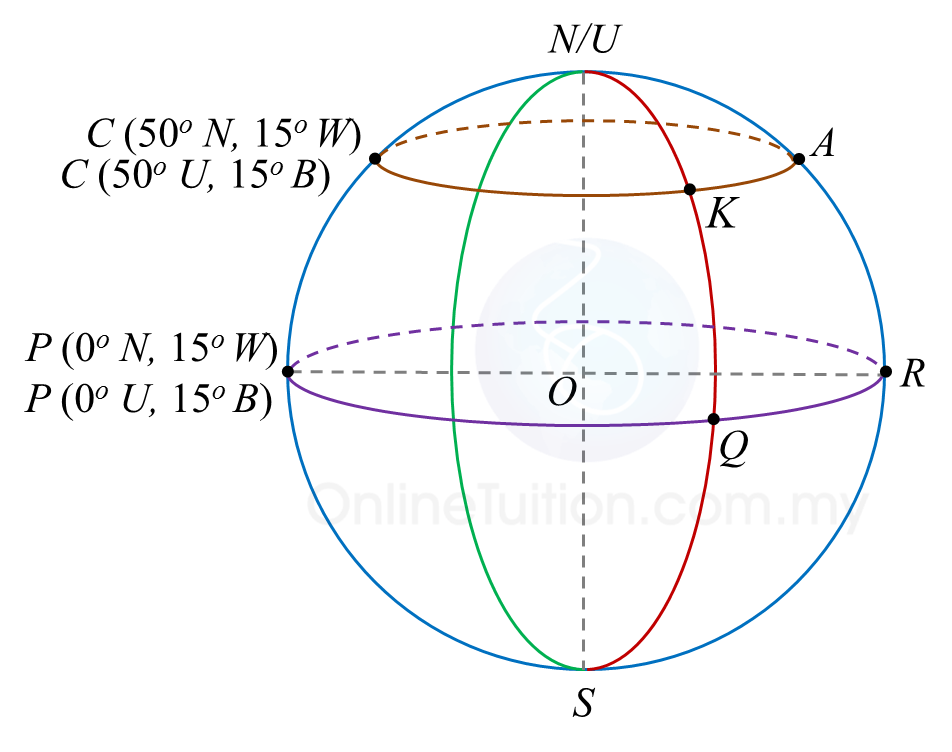# 9.6.3 Earth as a Sphere, SPM Paper 2 (Long Questions)

Question 5:
A (53o N, 84o E), B (53o N, 25o W), C and D are four points on the surface of the earth. AC is the diameter of the parallel of latitude 53o N.

(a)
State the location of C.

(b)
Calculate the shortest distance, in nautical mile, from A to C measured along the surface of the earth.

(c)
Calculate the distance, in nautical mile, from A due east B measured along the common parallel of latitude.

(d)
An aeroplane took off from B and flew due south to D. The average speed of the flight was 420 knots and the time taken was 6½ hours.
Calculate
(i) the distance, in nautical mile, from B to D measured along the meridian.
(ii) the latitude of D.

Solution:
(a)
Latitude of C = 53o N
Longitude of C = (180o – 84o) E = 96o E
Therefore location of C = (53o N, 96o E)

(b)
Shortest distance from A to C
= (180 – 53 – 53) x 60
= 74 x 60
= 4440 nautical miles

(c)
Distance from A to B
= (84 + 25) x 60 x cos 53o
= 109 x 60 x cos 53o
= 3935.87 nautical miles

(d)

Question 6:
Diagram below shows the locations of points P, Q, R, A, K and C, on the surface of the earth. O is the centre of the earth.(a) Find the location of A.

(b) Given the distance QR is 3240 nautical miles, find the longitude of Q.

(c) Calculate the distance, in nautical miles of KA, measured along the common parallel latitude.

(d) An aeroplane took off from A and flew due west to K along the common parallel of latitude. Then, it flew due south to Q. The average speed of the aeroplane was 550 knots.
Calculate the total time, in hours, taken for the whole flight.

Solution:
(a)
Longitude of A = (180o – 15o) = 165o E
Latitude of A = 50o N
Therefore, position of A = (50o N, 165o E).

(b)

(c)
Distance of KA
= 54 x 60 x cos 50o
= 2082.6 nautical miles

(d)

### 2 thoughts on “9.6.3 Earth as a Sphere, SPM Paper 2 (Long Questions)”

1.For queastion number 5 (c)
Why do you minus 84and 25?
Should it be plus? Because both are from different direction?
Thank you

•Hi,